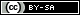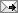## Gavin R. Putland,  BE PhD

 Monday, October 20, 1997 (Comment)

# Modeling of Horns and Enclosures for Loudspeakers (PhD thesis)

Author: Gavin R. Putland.  Institution: University of Queensland (Dept. of Electrical & Computer Engineering).  Supervisor: Dr. L. V. Skattebol.  Acceptance date: February 6, 1996.

The full text, incorporating the corrections listed at the end of this page, is available as a PDF file (1274kB, 22+293pp.).

Copyright © 1994–1997 Gavin Richard Putland: The thesis may be reproduced for the purposes of research or study with customary acknowledgment of the source.

### Abstract

It is shown that the “Webster” horn equation is an exact consequence of “one-parameter” or “1P” wave propagation. If a solution of the Helmholtz equation depends on a single spatial coordinate, that coordinate can be transformed to another coordinate, denoted by ξ, which measures arc length along the orthogonal trajectories to the constant-ξ surfaces. Webster's equation, with ξ as the axial coordinate, holds inside a tube of such orthogonal trajectories; the cross-sectional area in the equation is the area of a constant-ξ cross-section. This derivation of the horn equation makes no explicit assumption concerning the shape of the wavefronts. It is subsequently shown, however, that the wavefronts must be planar, circular-cylindrical or spherical, so that no new geometries for exact 1P acoustic waveguides remain to be discovered.

It is shown that if the linearized acoustic field equations are written in arbitrary curvilinear orthogonal coordinates and approximated by replacing all spatial derivatives by finite-difference quotients, the resulting equations can be interpreted as the nodal equations of a three-dimensional L-C network. This “finite-difference equivalent-circuit” or “FDEC” model can be truncated at the boundaries of the simulated region and terminated to represent a wide variety of boundary conditions. The presence of loosely-packed fibrous damping materials can be represented by using complex values for the density and ratio of specific heats of the medium. These complex quantities lead to additional components in the FDEC model.

Two examples of FDEC models are given. The first example predicts the frequency response of a moving-coil loudspeaker in a fiberglass-filled box, showing the effects of internal resonances. Variations of the model show how the properties of the fiberglass contribute to the damping of resonances and the shaping of the frequency response. It is found that viscosity, rather than heat conduction, is the dominant mechanism of damping. The second example addresses the classical problem of radiation from a circular rigid piston, and confirms that a free-air anechoic radiation condition with oblique incidence can be successfully represented in the FDEC model.

### Revision history

The thesis was accepted on February 6, 1996. The following corrections were made on February 9, 1997:

• After Eq. (8.62): “Putting x = 0” changed to “Putting x = 1”;
• Table 8.1, line 6: “Step until” changed to “Step(μ) until”;
• Line below Eq. (8.74): “μ1 = 247ms” changed to “τ1 = 247ms”;
• Bibliography: Page numbers added to reference .

The following corrections were made on October 20, 1997:

• Eq. (8.33): dS/dR changed to dS/dr ;
• Related Publications (page ix): Page numbers added.

The source files as revised on October 20, 1997, were converted to DVI format and thence to PDF using LaTeX2e and dvipdfm 0.12.6e, respectively. (These tools were distributed with version 1.20 of MiKTeX). In 2017, the title page was modified for uploading to hal.archives-ouvertes.fr.

The printed copy in the University of Queensland Library was made from a PostScript file in November 1995. The library version is the most recent that the author has proofread from start to finish. Its page numbers differ from those of the present PDF version.

[This page first published October 20, 1997; edited & relocated July 8, 2009; reformatted & relocated July 6, 2012; last modified March 16, 2020.]TweetReturn to Contents